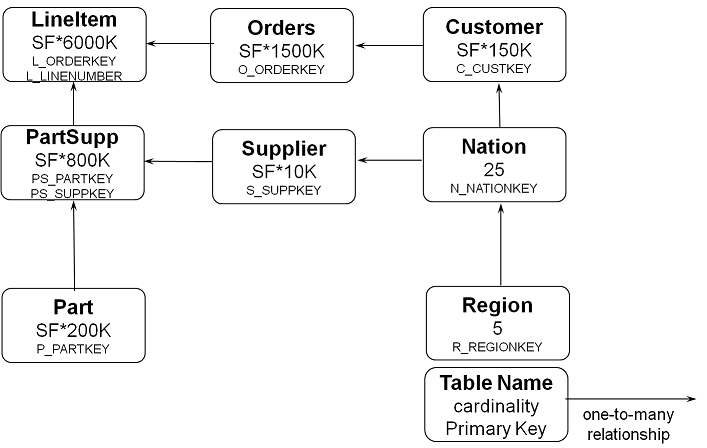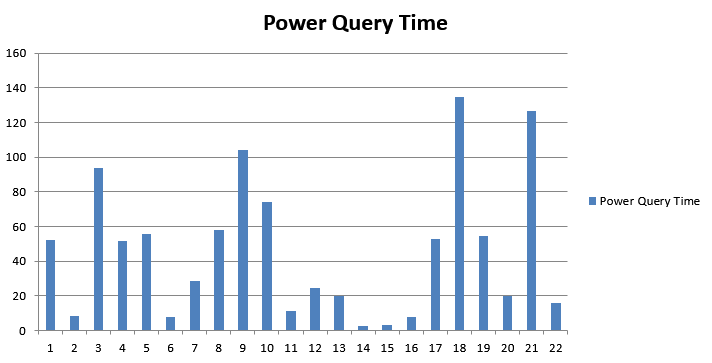## 1. What is TPROC-H derived from TPC-H?

Figure 11.1. TPROC-H Schema.The workload is represented by users executing a stream of 22 ad-hocs queries against the database with an example query as follows:

```-- using 647655760 as a seed to the RNG
select
l_returnflag,
l_linestatus,
sum(l_quantity) as sum_qty,
sum(l_extendedprice) as sum_base_price,
sum(l_extendedprice * (1 - l_discount)) as sum_disc_price,
sum(l_extendedprice * (1 - l_discount) * (1 + l_tax)) as sum_charge,
avg(l_quantity) as avg_qty,
avg(l_extendedprice) as avg_price,
avg(l_discount) as avg_disc,
count(*) as count_order
from
lineitem
where
l_shipdate <= date '1998-12-01' – interval '69' day (3)
group by
l_returnflag,
l_linestatus
order by
l_returnflag,
l_linestatus;
```

In measuring the results the key aspect is the time the queries take to complete and it is recommended to use the geometric mean of the query times for comparison. A typical performance profile is represented by the time it takes the system to process a query set from Q1 to Q22 (run in a pre-determined random order).

Figure 11.2. Power Query